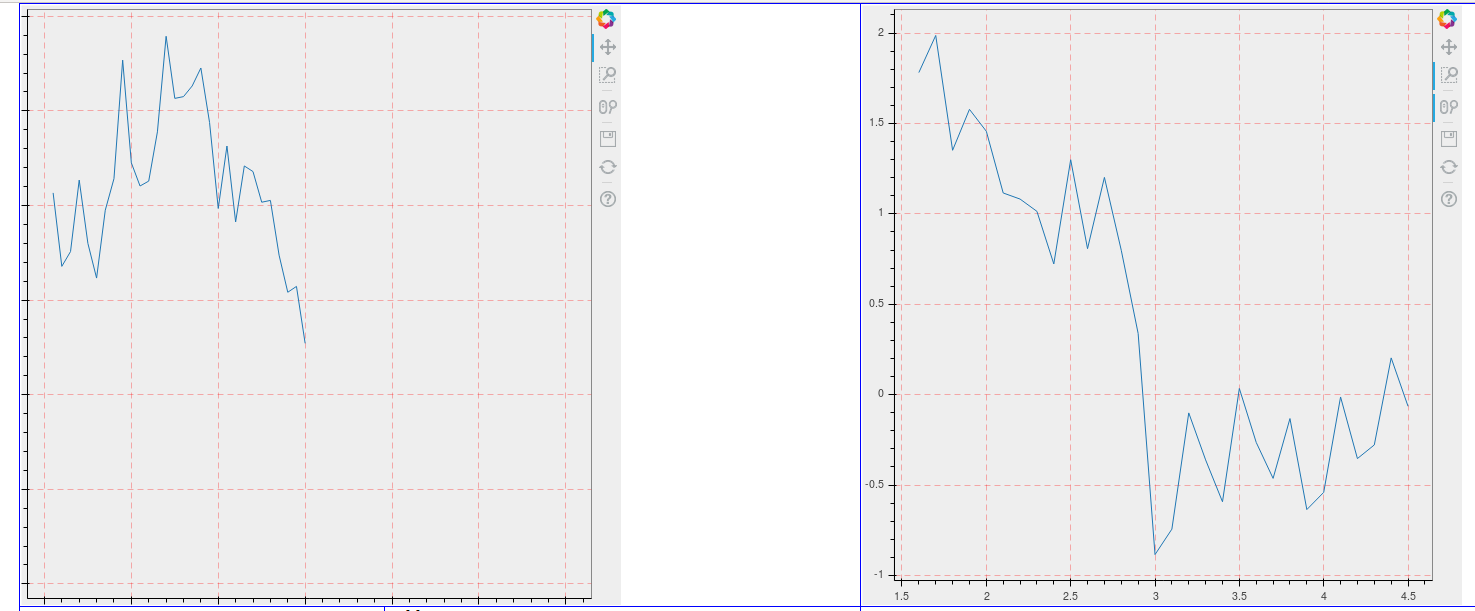# Missing numbers in embed graphs

I’m embedding a couple of streaming plots.
Using Pythin 2.7 and Bokeh 0.12.6.

The code is:

``````
p = figure()
p.x_range.follow_interval = 10
p.axis.visible = True

source1 = AjaxDataSource(data=dict(x1=[], y1=[]),
data_url='http://127.0.0.1:5050/data/1',
polling_interval=300)

p.line(x='x1', y='y1', source=source1)
script1, div1 = components(p)
``````

Is there a reason why the scale numbers are not visible and then they become visible only after pressing Reset?
Axis and ticks are always visible.

In the image two plots: on the left as they are at start, on the right after pressing Reset.Hi,

The latest release fixed many layout-related bugs, but there is still work to do. I'm guessing this is a bug, perhaps related to this one:

I've not specifically encountered this situation with a standard plot, though. you might try increasing the value of "min_border" on the plot.

Thanks,

Bryan

···

On Jun 23, 2017, at 02:44, Alessandro Pocaterra <[email protected]> wrote:

I'm embedding a couple of streaming plots.
Using Pythin 2.7 and Bokeh 0.12.6.

The code is:

p = figure()
p.x_range.follow_interval = 10
p.axis.visible = True

source1 = AjaxDataSource(data=dict(x1=, y1=),
data_url='http://127.0.0.1:5050/data/1',
polling_interval=300)

p.line(x='x1', y='y1', source=source1)
script1, div1 = components(p)

Is there a reason why the scale numbers are not visible and then they become visible only after pressing Reset?
Axis and ticks are always visible.

In the image two plots: on the left as they are at start, on the right after pressing Reset.

--
You received this message because you are subscribed to the Google Groups "Bokeh Discussion - Public" group.
To unsubscribe from this group and stop receiving emails from it, send an email to [email protected].
To post to this group, send email to [email protected].
To view this discussion on the web visit https://groups.google.com/a/continuum.io/d/msgid/bokeh/2417550a-4ae2-40a8-8079-7851e4f91453%40continuum.io.
<Screenshot from 2017-06-23 09-38-02.png>

Hi Brian.
I found by chance a trick to solve the issue:

``````AjaxDataSource(data=dict(x1=, y1=), ...
``````
···

The 1 added between [ and ] is enough to show the numbers immediately.

Thank you

Alex

On Jul 1, 2017 9:55 PM, “Bryan Van de ven” [email protected] wrote:

Hi,

The latest release fixed many layout-related bugs, but there is still work to do. I’m guessing this is a bug, perhaps related to this one:

``````    [https://github.com/bokeh/bokeh/issues/6356](https://github.com/bokeh/bokeh/issues/6356)
``````

I’ve not specifically encountered this situation with a standard plot, though. you might try increasing the value of “min_border” on the plot.

Thanks,

Bryan

On Jun 23, 2017, at 02:44, Alessandro Pocaterra [email protected] wrote:

I’m embedding a couple of streaming plots.

Using Pythin 2.7 and Bokeh 0.12.6.

The code is:

p = figure()

p.x_range.follow_interval = 10

p.axis.visible = True

source1 = AjaxDataSource(data=dict(x1=, y1=),

``````                    data_url='[http://127.0.0.1:5050/data/1](http://127.0.0.1:5050/data/1)',
``````
``````                    polling_interval=300)
``````

p.line(x=‘x1’, y=‘y1’, source=source1)

script1, div1 = components§

Is there a reason why the scale numbers are not visible and then they become visible only after pressing Reset?

Axis and ticks are always visible.

In the image two plots: on the left as they are at start, on the right after pressing Reset.

Hi,

Seems like a weird bug/corener case. Please feel free to file a GitHub issue with this information.

Thanks,

Bryan

···

On Jul 10, 2017, at 18:41, Alessandro Pocaterra <[email protected]> wrote:

Hi Brian.
I found by chance a trick to solve the issue:
AjaxDataSource(data=dict(x1=, y1=), ...
The 1 added between [ and ] is enough to show the numbers immediately.

Thank you
Alex

On Jul 1, 2017 9:55 PM, "Bryan Van de ven" <[email protected]> wrote:
Hi,

The latest release fixed many layout-related bugs, but there is still work to do. I'm guessing this is a bug, perhaps related to this one:

I've not specifically encountered this situation with a standard plot, though. you might try increasing the value of "min_border" on the plot.

Thanks,

Bryan

> On Jun 23, 2017, at 02:44, Alessandro Pocaterra <[email protected]> wrote:
>
> I'm embedding a couple of streaming plots.
> Using Pythin 2.7 and Bokeh 0.12.6.
>
> The code is:
>
> p = figure()
> p.x_range.follow_interval = 10
> p.axis.visible = True
>
> source1 = AjaxDataSource(data=dict(x1=, y1=),
> data_url='http://127.0.0.1:5050/data/1',
> polling_interval=300)
>
> p.line(x='x1', y='y1', source=source1)
> script1, div1 = components(p)
>
> Is there a reason why the scale numbers are not visible and then they become visible only after pressing Reset?
> Axis and ticks are always visible.
>
> In the image two plots: on the left as they are at start, on the right after pressing Reset.
>
> --

--
You received this message because you are subscribed to the Google Groups "Bokeh Discussion - Public" group.
To unsubscribe from this group and stop receiving emails from it, send an email to [email protected].
To post to this group, send email to [email protected].
To view this discussion on the web visit https://groups.google.com/a/continuum.io/d/msgid/bokeh/CAO4uU9O9iibK0p7e88z2%3D4ymtpF4EZ61TV_xLToJ2mjzXc2a2g%40mail.gmail.com.